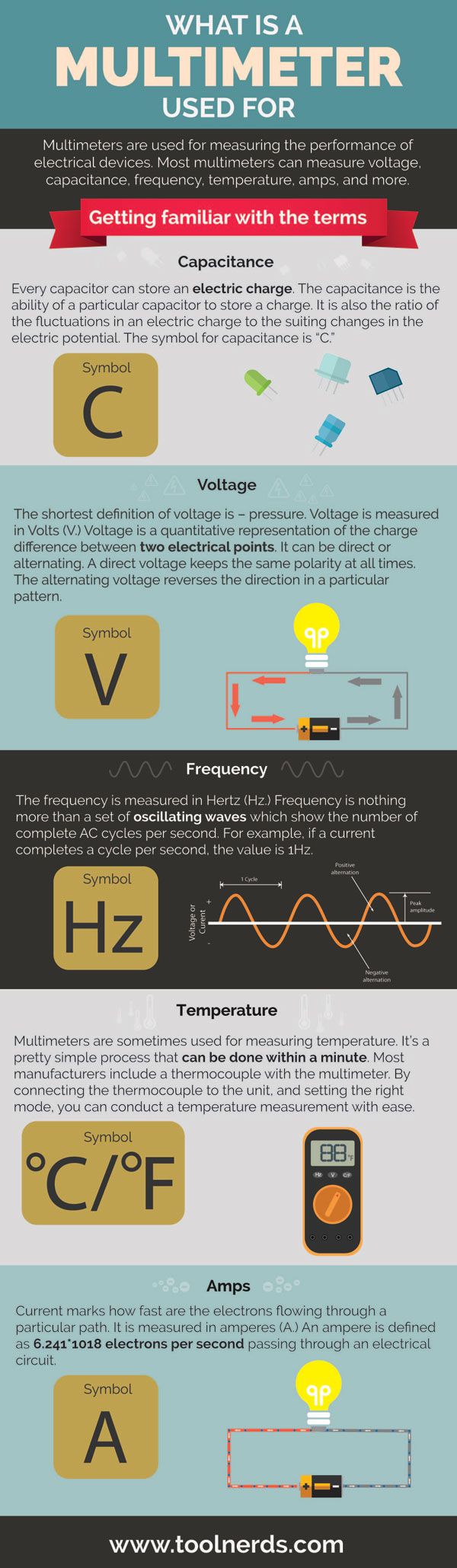Multimeters are used for measuring the performance of electrical devices. Most multimeters can measure voltage, capacitance, frequency, temperature, amps, and more.

## Getting Familiar with the Terms

### Capacitance

Every capacitor can store an electric charge. The capacitance is the ability of a particular capacitor to store a charge. It is also the ratio of the fluctuations in an electric charge to the suiting changes in the electric potential. The symbol for capacitance is “C.”

### Voltage

The shortest definition of voltage is – pressure. Voltage is measured in Volts (V). Voltage is a quantitative representation of the charge difference between two electrical points. It can be direct or alternating. A direct voltage keeps the same polarity at all times. The alternating voltage reverses the direction in a particular pattern.

### Frequency

The frequency is measured in Hertz (Hz). Frequency is nothing more than a set of oscillating waves which show the number of complete AC cycles per second. For example, if a current completes a cycle per second, the value is 1Hz.

### Temperature

Multimeters are sometimes used for measuring temperature. It’s a pretty simple process that can be done within a minute. Most manufacturers include a thermocouple with the multimeter. By connecting the thermocouple to the unit, and setting the right mode, you can conduct a temperature measurement with ease.

### Amps

Current marks how fast are the electrons flowing through a particular path. It is measured in amperes (A). An ampere is defined as 6.241*1018 electrons per second passing through an electrical circuit.